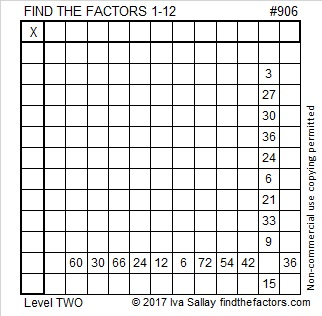# 906 and Level 2

906 looks the same upside-down so it is a strobogrammatic number.

We can tell just by looking at 906 that it is divisible by 1, 2, and 3. That means it can also be evenly divided by 6.

906 is the sum of consecutive prime numbers: 449 + 457 = 906

It is also palindrome 636 in BASE 12 because 6(144) + 3(12) + 6(1) = 906.Print the puzzles or type the solution on this excel file: 12 factors 905-913

• 906 is a composite number.
• Prime factorization: 906 = 2 × 3 × 151
• The exponents in the prime factorization are 1, 1, and 1. Adding one to each and multiplying we get (1 + 1)(1 + 1)(1 + 1) = 2 × 2 × 2 = 8. Therefore 906 has exactly 8 factors.
• Factors of 906: 1, 2, 3, 6, 151, 302, 453, 906
• Factor pairs: 906 = 1 × 906, 2 × 453, 3 × 302, or 6 × 151
• 906 has no square factors that allow its square root to be simplified. √906 ≈ 30.099833887This site uses Akismet to reduce spam. Learn how your comment data is processed.